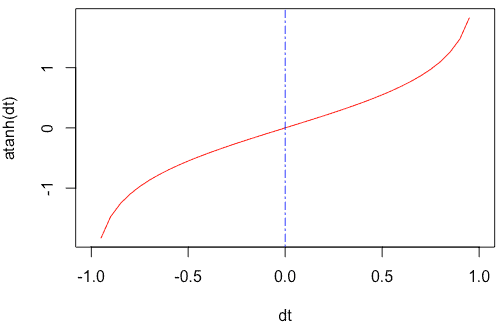# What is the atanh() Function in R

The atanh() function in R is “used to calculate the inverse hyperbolic tangent of a number”. For real numbers, -1 < x < 1. For complex numbers, the range is -Inf < x < -1 and 1 < x < Inf.

### Syntax

``atanh(x)``

### Parameters

x: It is a numeric value, array, or vector.

## Example 1: How to use the atanh() function

``atanh(1)``

Output

`` Inf``

If you pass the 0 to the atanh() function, it will return 0.

``atanh(0)``

Output

`` 0``

## Example 2: Calculating atanh() of a complex number

``````d <- 5 + 1i
atanh(d)``````

Output

`` 0.194426+1.530881i``

## Example 3: Using atanh() function to a Vector

``````rv <- c(-1, 0.5, 0, 0.5, 1)
atanh(rv)``````

Output

`` -Inf 0.5493061 0.0000000 0.5493061 Inf``

## Example 4: Passing a pi to the atanh() function

``atanh(pi)``

Output

`````` NaN
Warning message:
In atanh(pi) : NaNs produced``````

Let’s see another example of pi.

``atanh(pi / 4)``

Output

`` 1.059306``

## Plot the atanh() function to a graph

We can use the seq() function to create a series of values and pass that to the plot() function, creating a line chart.

``````dt <- seq(-1, 1, by = 0.05)

plot(dt, atanh(dt), typ = "l", col = "red")

abline(v = 0, lty = 6, col = "blue")``````

Output## Other Hyperbolic Trigonometric Functions

R cosh(T x)

The hyperbolic cosine of x (in radians).

The hyperbolic sine of x (in radians).

The hyperbolic tangent of x (in radians).

It is an inverse hyperbolic cosine (in radians).

It is an inverse hyperbolic cosine (in radians).

R atanh(T x)

It is an inverse hyperbolic tangent (in radians) of x.

That is it.